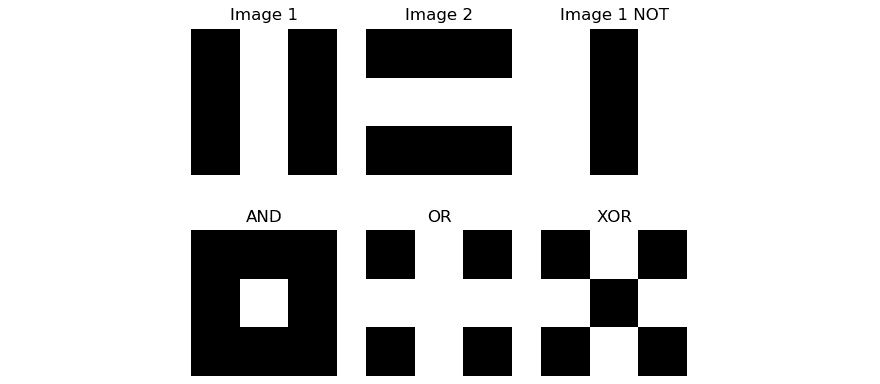• 检索图像属性
• 图像的基本操作
• 图像上的算术运算
• 融合和过渡图像
• 将图像与常数相乘
• 创建图像底片
• 图像上的按位逻辑运算

# 检索图像属性

>>> import cv2
>>> print(type(img))


<class 'numpy.ndarray'>


>>> print(img.ndim)
2


>>> print(img.shape)
(256, 256)


>>> print(img.size)
65536


>>> print(img.dtype)
uint8


>>> print(img.nbytes)
65536


>>> img = cv2.imread('/home/pi/book/dataset/4.1.01.tiff', 1)
>>> print(type(img))


<class 'numpy.ndarray'>


>>> print(img.ndim)
3


>>> print(img.shape)
(256, 256, 3)


>>> print(img.size)
196608


>>> print(img.dtype)
uint8


>>> print(img.nbytes)
196608


# 图像的基本操作

>>> import cv2


>>> print(img[10, 10])
[34 38 44]


>>> print(img[10, 10, 0])
34


## 将图像分成通道

>>> import cv2
>>> b, g, r = cv2.split(img)


>>> b = img[:, :, 0]
>>> g = img[:, :, 1]
>>> r = img[:, :, 2]


split()函数（从计算上来说）比以前的 NumPy 索引方法要昂贵一些。 我们还可以合并渠道，如下所示：

>>> img1 = cv2.merge((b, g, r))


## 为图像添加边框

• src：图像
• topbottomleftright：以像素数表示的边框宽度
• borderType: 边框的类型。 可以是以下类型之一：

a）cv2.BORDER_REFLECT

b）cv2.BORDER_REFLECT_101cv2.BORDER_DEFAULT

c）cv2.BORDER_REPLICATE

d）cv2.BORDER_WRAP

e）cv2.BORDER_CONSTANT：添加具有恒定颜色的边框。 边框颜色的值是以下参数。

• value：边框类型为cv2.BORDER_CONSTANT时边框的颜色

import cv2
b1 = cv2.copyMakeBorder(img, 10, 10, 10, 10, cv2.BORDER_WRAP)
b2 = cv2.copyMakeBorder(img, 10, 10, 10, 10, cv2.BORDER_CONSTANT, value=[255, 0, 0])
cv2.imshow('Wrap', b1)
cv2.imshow('Constant', b2)
cv2.waitKey(0)
cv2.destroyAllWindows()cv2.copyMakeBorder(img, 10, 10, 10, 10, cv2.BORDER_REPLICATE)


cv2.copyMakeBorder(img, 10, 10, 10, 10, cv2.BORDER_REFLECT)
cv2.copyMakeBorder(img, 10, 10, 10, 10, cv2.BORDER_REFLECT_101)


# 图像的算术运算

import cv2
cv2.imshow('NumPy Addition', img1 + img2 )
cv2.waitKey(0)
cv2.destroyAllWindows()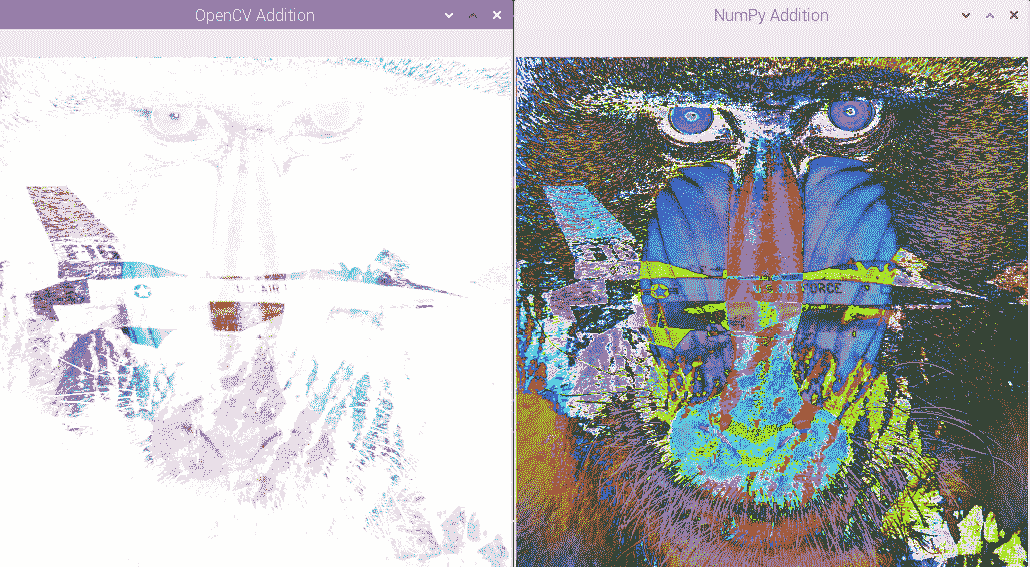>>> import numpy as np
>>> import cv2
>>> a = np.array(, np.uint8)
>>> b = np.array(, np.uint8)
>>> a + b
array(, dtype=uint8)


>>> cv2.add(a, b)
array([], dtype=uint8)


import cv2
cv2.imshow('NumPy Subtract', img1 - img2)
cv2.imshow('OpenCV Subtract', cv2.subtract(img1, img2))
cv2.waitKey(0)
cv2.destroyAllWindows()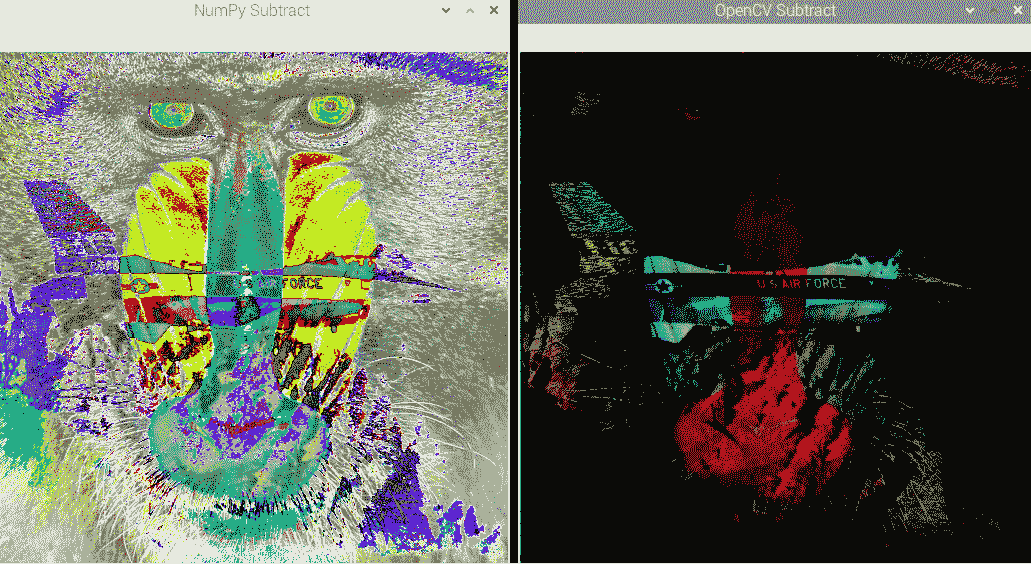>>> import cv2
>>> import numpy as np
>>> a = np.array(, np.uint8)
>>> b = np.array(, np.uint8)
>>> b - a
array(, dtype=uint8)


>>> cv2.subtract(b, a)
array([], dtype=uint8)


# 融合和过渡图像

cv2.addWeighted()函数计算我们将作为参数传递的两个图像的加权和。 这导致它们融合。 以下是演示此混合概念的一些代码：

import cv2
cv2.imshow('Blended Image',
cv2.waitKey(0)
cv2.destroyAllWindows()


• img1：第一张图片
• alpha：第一张图片的系数（在前面的示例中为0.5
• img2：第二张图片
• beta：第二张图片的系数（在前面的示例中为0.5
• gamma：标量值（在前面的示例中为0

OpenCV 使用以下公式来计算输出图像：

输出图像 = (alpha * img1) + (beta * img2) + gamma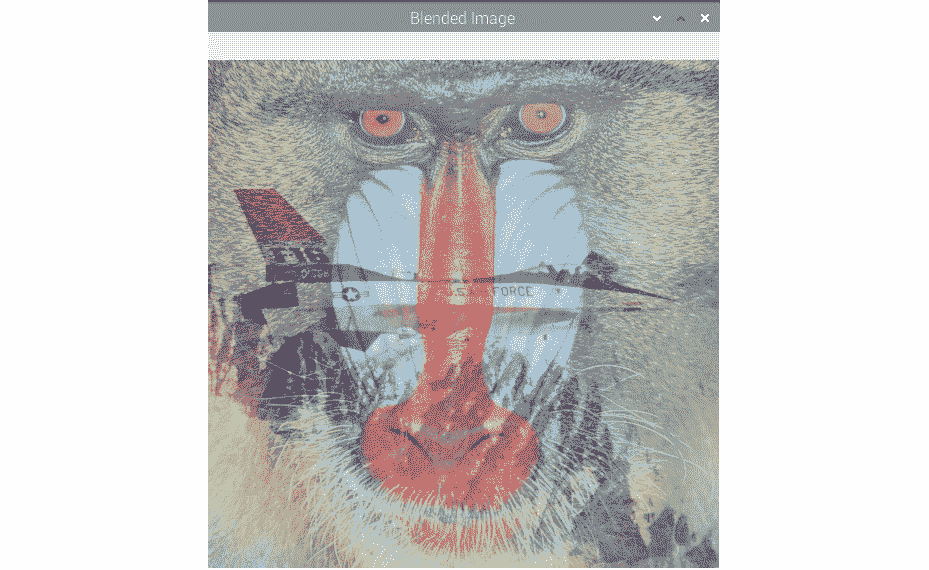import cv2
import time
import numpy as np
for i in np.linspace(0, 1, 100):
alpha = i
beta = 1-alpha
print('ALPHA =' + str(alpha) + ' BETA =' + str(beta))
cv2.imshow('Image Transition',
time.sleep(0.05)
if cv2.waitKey(1) == 27 :
break
cv2.destroyAllWindows()


import cv2
import time
import numpy as np
def emptyFunction():
pass
output = cv2.addWeighted(img1, 0.5, img2, 0.5, 0)
windowName = "Transition Demo"
cv2.namedWindow(windowName)
cv2.createTrackbar('Alpha', windowName, 0,
1000, emptyFunction)
while(True):
cv2.imshow(windowName, output)
if cv2.waitKey(1) == 27:
break
alpha = cv2.getTrackbarPos('Alpha', windowName) / 1000
beta = 1 - alpha
output = cv2.addWeighted(img1, alpha, img2, beta, 0)
print(alpha, beta)
cv2.destroyAllWindows()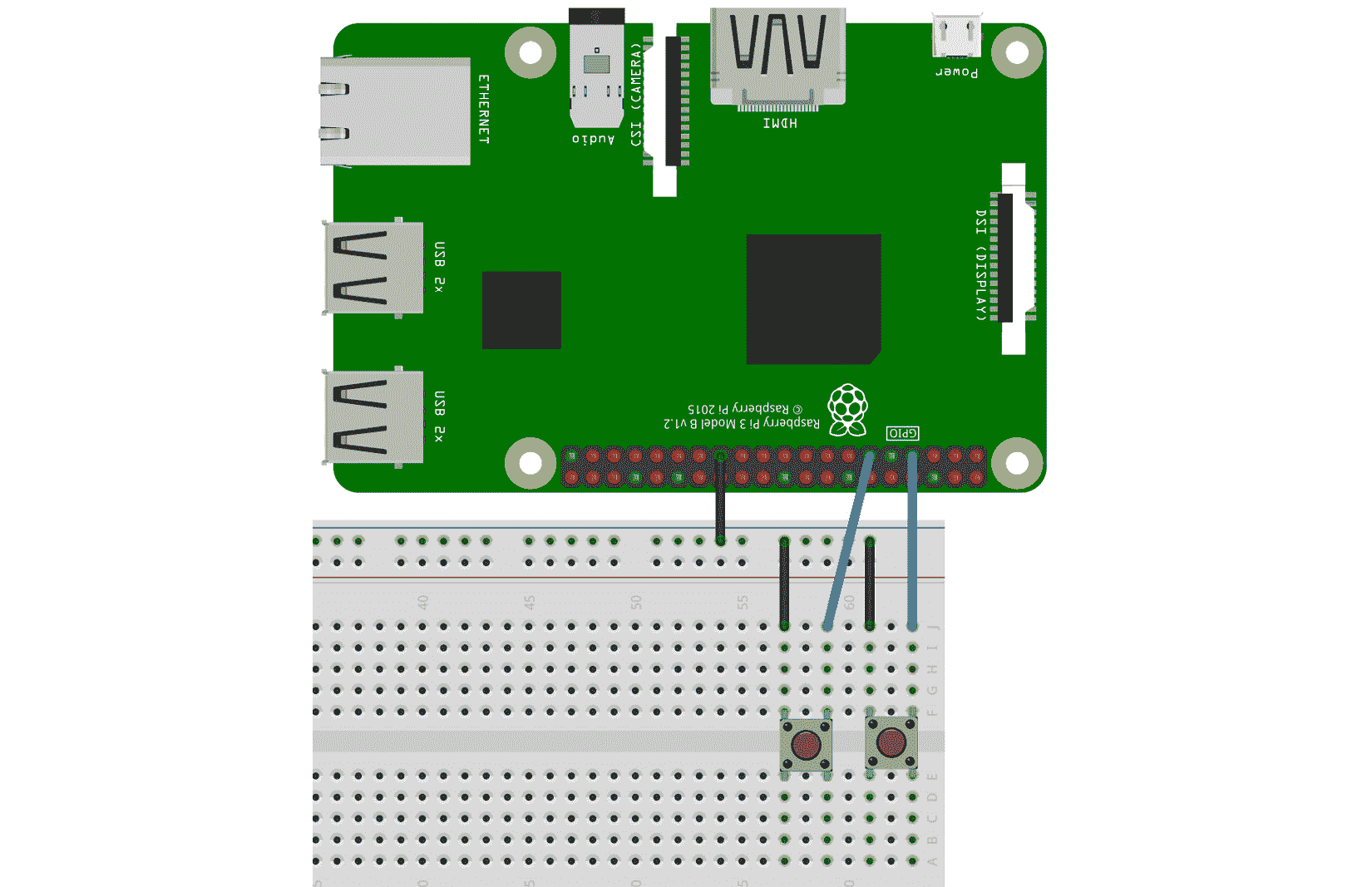import time
import RPi.GPIO as GPIO
import cv2
alpha = 0
GPIO.setmode(GPIO.BOARD)
GPIO.setwarnings(False)
button1 = 7
button2 = 11
GPIO.setup(button1, GPIO.IN, GPIO.PUD_UP)
GPIO.setup(button2, GPIO.IN, GPIO.PUD_UP)
while True:
button1_state = GPIO.input(button1)
if button1_state == GPIO.LOW and alpha < 1:
alpha = alpha + 0.2
button2_state = GPIO.input(button2)
if button2_state == GPIO.LOW:
if (alpha > 0):
alpha = alpha - 0.2
if (alpha < 0):
alpha = 0
beta = 1 - alpha
output = cv2.addWeighted(img1, alpha, img2, beta, 0)
cv2.imshow('Transition App', output)
if cv2.waitKey(1) == 27:
break
time.sleep(0.5)
print(alpha)
cv2.destroyAllWindows()


# 将图像乘以一个常数

import cv2
cv2.imshow('Image1', img1 * 2)
cv2.waitKey(0)
cv2.destroyAllWindows()


cv2.imshow('Image1', img1 * 2)


# 创建图像底片

import cv2
negative = abs(255 - img)
cv2.imshow('Grayscale', img)
cv2.imshow('Negative', negative)
cv2.waitKey(0)
cv2.destroyAllWindows()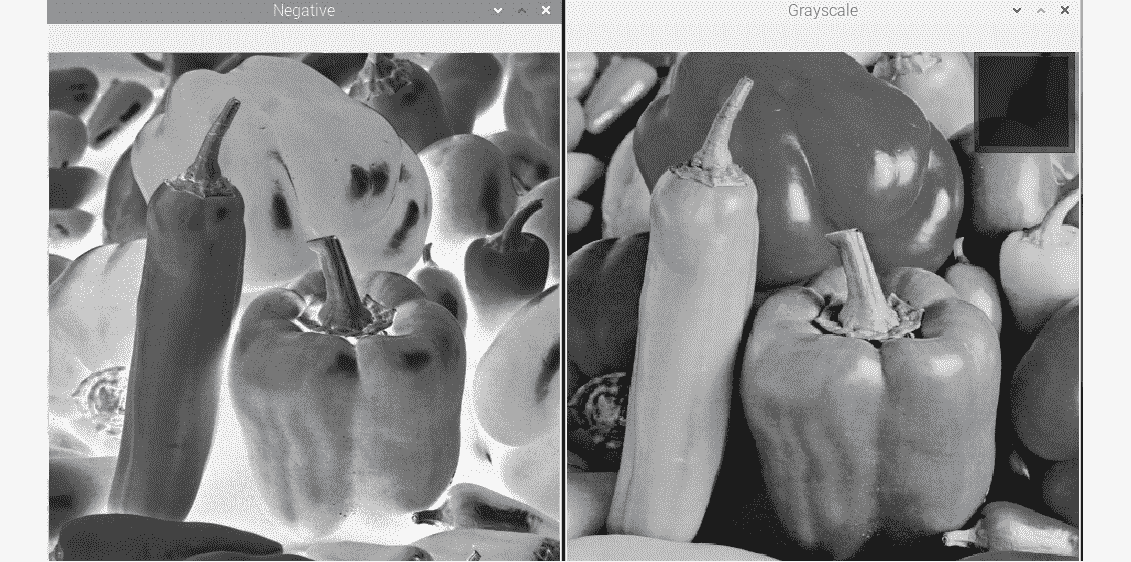# 对图像进行按位逻辑运算

OpenCV 库具有许多用于计算图像上按位逻辑运算的功能。 我们可以计算按位逻辑异或XOR），（反转）操作。 演示这些功能如何工作的最佳方法是将其与二进制（黑白）图像一起使用：

import cv2
import numpy as np
import matplotlib.pyplot as plt
a = [0, 255, 0]
img1 = np.array([a, a, a], dtype=np.uint8)
img2 = np.transpose(img1)
not_out = cv2.bitwise_not(img1 )
and_out = cv2.bitwise_and(img1, img2)
or_out = cv2.bitwise_or(img1, img2)
xor_out = cv2.bitwise_xor(img1, img2)
titles = ['Image 1', 'Image 2', 'Image 1 NOT', 'AND', 'OR', 'XOR']
images = [img1, img2, not_out, and_out, or_out, xor_out]
for i in range(6):
plt.subplot(2, 3, i+1)
plt.imshow(images[i], cmap='gray')
plt.title(titles[i])
plt.axis('off')
plt.show()


plt.subplot(2, 3, 1)
plt.imshow(img1, cmap='gray')
plt.title('Image 1')
plt.axis('off')